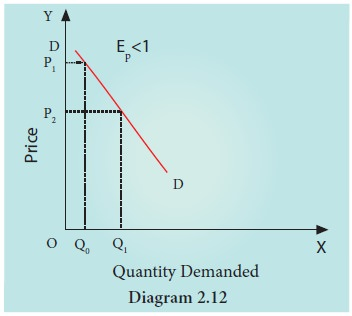Home | | Economics 11th std | Levels or Degrees of Price Elasticity of Demand

# Levels or Degrees of Price Elasticity of Demand

The Price Elasticity of Demand is commonly known as the elasticity of demand, which refers to the degree of responsiveness of demand to the change in the price of the commodity.

Levels or Degrees of Price Elasticity of Demand

Definition: The Price Elasticity of Demand is commonly known as the elasticity of demand, which refers to the degree of responsiveness of demand to the change in the price of the commodity.

## 1. Perfectly Elastic Demand (Ep = ∞):The demand is said to be perfectly elastic when a slight change in the price of a commodity causes an infinite change in its quantity demanded. Such as, even a small rise in the price of a commodity can result in greater fall in demand even to zero. In some cases a little fall in the price can result in the increase in demand to infinity. In perfectly elastic demand the demand curve is a horizontal straight line parallel to x axis.

## 2. Perfectly Inelastic Demand (Ep =0):

When there is no change in the demand for a product due to the change in the price, then the demand is said to be perfectly inelastic. Here, the demand curve is a vertical straight line which shows that the demand remains unchanged irrespective of change in the price., i.e. quantity OQ remains unchanged at different prices, P1, P2, and P3.## 3. Relatively Elastic Demand (Ep>1):The demand is relatively elastic when the proportionate change in the demand for a commodity is greater than the proportionate change in its price. Here, the demand curve is gradually sloping which shows that a proportionate change in quantity from 5 to 10 is greater than the proportionate change in the price from 11 to 10. Change in demand is:

10–5/5 × 100 = 100%

Change in price =10%. Hence, it is more elastic demand.

## 4. Relatively Inelastic Demand (Ep<1):

When the proportionate change in the demand for a product is less than the proportionate change in the price, the demand is said to be relatively inelastic.It is also called as the elasticity less than unity. Here the demand curve is steeply sloping, which shows that the change in the quantity from OQ0 to OQ1 is relatively smaller than the change in the price from OP1 to Op2.

## 5. Unitary Elastic Demand (Ep =1):

The demand is unitary elastic when the proportionate change in the price of a product results in the same propionate change in the quantity demanded. Here the shape of the demand curve is a rectangular hyperbola, which shows that area under the curve is equal to one.Here OP0 R0Q0 = OP1 R1Q1Tags : Economics , 11th Economics : Chapter 2 : Consumption Analysis
Study Material, Lecturing Notes, Assignment, Reference, Wiki description explanation, brief detail
11th Economics : Chapter 2 : Consumption Analysis : Levels or Degrees of Price Elasticity of Demand | Economics